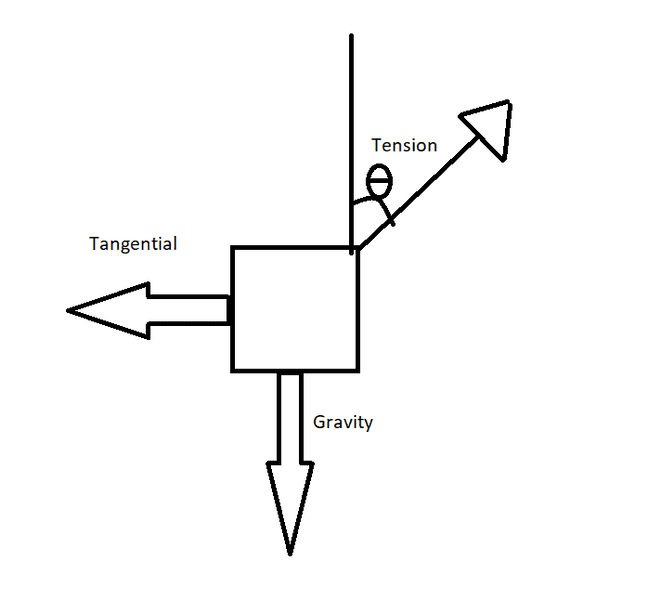# Tangential Acceleration/Tension

I did the problem, just want to validate my solution/approach

1. Homework Statement

Your car happens to have a fuzzy die hanging from the rear view mirror. As you round a curve that is approximately a circle of radius R you notice that the fuzzy die makes an angle θ with the vertical. What is the angle θ as a function of R and your speed v?

## Homework Equations

$$\sum F = ma$$
$$F = \frac{mv^2}{r}$$

## The Attempt at a Solution

There are 3 forces on the dice as shown in the free body diagram attachmentFrom this the sum of the vertical forces and horizontal forces must cancel out, so we can say
$$F_{\text{tension,y}} = F_{\text{gravity}}$$
$$F_{\text{tension}} \cdot cos \theta = F_{\text{gravity}}$$
$$F_{\text{tension}} \cdot cos \theta =mg$$
$$F_{\text{tension}}= \frac{mg}{cos \theta}$$
and
$$F_{\text{tension,x}} = F_{\text{tangential}}$$
$$F_{\text{tension}} \cdot sin \theta = F_{\text{tangential}}$$
$$F_{\text{tension}} \cdot sin \theta = \frac{mv^2}{r}$$
$$\frac{mv^2}{r} = mg \cdot \frac{sin \theta}{cos \theta}$$
$$tan \theta = \frac{v^2}{rg}$$
$$\theta = tan^{-1}\frac{v^2}{rg}$$

#### Attachments

Last edited:

Orodruin
Staff Emeritus
Homework Helper
Gold Member
2021 Award
Your idea is fine but you made an error in solving for ##\tan(\theta)## (check the units, you cannot take the arctan of a dimensionful number!). Alternatively you can immediately conclude that the tension force, gravity, and tangential force must form a right triangle where the tension force is the hypothenuse, the gravitational force is the side closest to the angle ##\theta##, and the tangential force the far side. It directly follows that
$$\tan(\theta) = \frac{F_{\rm tang}}{F_g} = \ldots$$
(I am leaving the dots for you to get the correct expression yourself)

Edit: Note that ##gv^2/r## has dimension ##\mathsf{L^2/T^4}##, i.e., not dimensionless.

srekai: Your solution is correct. v^2 / rg is in fact dimensionless.

Orodruin
Staff Emeritus
Homework Helper
Gold Member
2021 Award
srekai: Your solution is correct. v^2 / rg is in fact dimensionless.
He obviously edited that after my post.

Chandra Prayaga
I have one remark and one question.
1. The component F sinθ should be in the radial direction, not tangential. It is pointing towards the center of the circle.
2. What is exerting the tangential force? I see only two forces, the tension and gravity.

haruspex
Homework Helper
Gold Member
I have one remark and one question.
1. The component F sinθ should be in the radial direction, not tangential. It is pointing towards the center of the circle.
2. What is exerting the tangential force? I see only two forces, the tension and gravity.
All that is wrong in @srekai's (edited) solution is the reference to "tangential" force. A tangential force would be normal to the string, and as you say there is no such force present. But if we label it centrifugal force instead it all works.

Chandra Prayaga